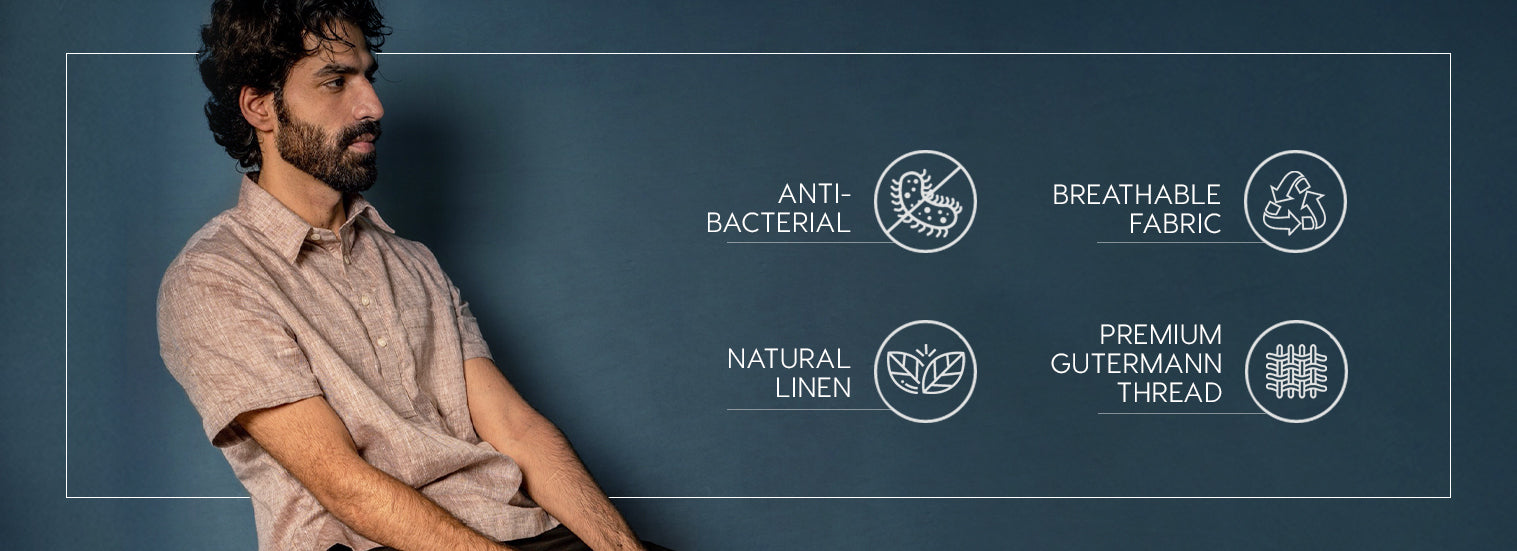Filter[49 products]
[49 products]
Rs. 4,499
Rs. 4,499
Rs. 4,499
Rs. 4,499
Rs. 4,499
Rs. 4,499
Rs. 4,999
Rs. 4,499
Rs. 4,499
Rs. 4,499
Rs. 4,499
Rs. 4,499
Rs. 4,499
Rs. 4,999
Rs. 4,499
Rs. 4,499
Rs. 4,499
Rs. 4,499
Rs. 4,499
Rs. 4,499
Rs. 4,999
Rs. 4,499
Rs. 4,499
Rs. 4,499
Rs. 4,499
Rs. 4,499
Rs. 4,499
Rs. 4,999
Rs. 4,499
Rs. 4,499
Rs. 4,499
Rs. 4,499
Rs. 4,499
Rs. 4,499
Rs. 4,999
Rs. 4,499
Rs. 4,499
Rs. 4,499
Rs. 4,499
Rs. 4,499
Rs. 4,499
Rs. 4,999
Rs. 4,499
Rs. 4,999
Rs. 4,999
Rs. 4,999
Rs. 4,999
Rs. 4,999
Rs. 4,999